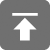# 工学1号馆

home

## C安全编码–整数理解

Wu Yudong    September 11, 2016     C   834

## 建议和规则

• 理解编译器所使用的数据模型
• 使用rsize_t或size_t类型表示所有表示对象长度的整数值
• 理解整数转换规则
• 使用安全的整数库
• 对来自不信任来源的整数值实行限制
• 如果输入函数无法处理所有可能出现的错误就不要用它们转换字符数据
• 使用strtol()或相关函数把字符串标记换换为整数
• 只使用显式的有符号或无符号char类型表示数值
• 验证所有的整数值位于范围内
• 保证枚举常量映射到唯一的值
• 使用%操作符时不要假设余数总是正的
• 把指针转换为整数或者把整数转换为指针时需要小心
• 当普通的整数位段用于表达式时，不要对它的类型作出假设
• 只对无符号操作数使用位操作符
• 避免在同一个数据上执行位操作和算术运算
• 在程序员定义的整数类型的格式化I/O中使用intmax_t或者unitmax_t

• 保证无符号整数运算不产生回绕
• 保证整型转换不会丢失或错误解释数据
• 保证有符号整数运算不会产生溢出
• 保证除法和求模运算不会导致除零错误
• 移位的数量不能是负数或大于操作数的位数
• 把整型表达式比较或赋值为一种较大类型之前用这种较大类型对它进行求值

## 理解编译器所使用的数据模型

<limits.h>除了提供特定类型的位数信息之外，还定义了一些宏，可以用于确定任何遵循标准的编译器所使用的标准整数类型数的整型范围

e.g.  UNIT_MAX是unsigned int可以出现的最大值，LONG_MIN是long int可以出现的最小值

<sdtint.h>头文件在类型中引入了特定的长度限制，可用于避免对特定数据模型的依赖

e.g.  int_least32_t是编译器所支持的最小有符号整数类型，它包含了至少32位<inttypes.h>声明了用于操纵最大宽度整数以及把数值字符串转换为最大宽度整数的函数

unsigned int a, b;
unsigned long c;
//初始化a和b
c = (unsigned long)a * b;  //可能会溢出

#if UNIT_MAX > UNITMAX / UNIT_MAX
#error No safe type is available.
#endif
unsigned int a, b;
unsigned long c;
//初始化a和b
c = (unitmax_t)a * b;

## 使用rsize_t或size_t类型表示所有表示对象长度的整数值

size_t类型表示sizeof操作符执行结果的无符号整型类型。size_t类型的变量保证具有足够的精度，能够表示一个对象的长度。2007年引入了一种新的类型rsize_t，它被定义为size_t，但是明确用于保存单个对象的长度，任何表示对象长度的变量，包括作为大小、索引、循环计数器和长度的整数值，如果有可能，都应该声明为rsize_t，否则就声明为size_t

char *copy(size_t n, const char *str) {
int i;
char *p;
if(n == 0) {
//error...
}
p = (char*)malloc(n);
if(p == NULL) {
//error...
}
for(i = 0; i < n; ++i) {
p[i] = *str++;
}
return p;
}
char *p = copy(9, "hi there");

char *copy(rsize_t n, const char *str) {
int i;
char *p;
if(n == 0 || n > RSIZE_MAX) {
//error...
}
p = (char*)malloc(n);
if(p == NULL) {
//error...
}
for(i = 0; i < n; ++i) {
p[i] = *str++;
}
return p;
}
char *p = copy(9, "hi there");

## 理解整数转换规则

#include<stdio.h>
int main(void){
signed char cresult, c1, c2, c3;
c1 = 100;
c2 = 3;
c3 = 4;
cresult = c1 * c2 / c3;
printf("cresult= %d\n", cresult);  //cresult= 75
return 0;
}

## 对来自不信任来源的整数值实行限制

int create_table(size_t length) {
char **table;
if(sizeof(char *) > SIZE_MAX / length) {
return -1; //溢出
}
size_t table_length = length * sizeof(char*);
table = (char**)malloc(table_length);
if(table == NULL) {
return -1; //error
}
/*...*/
return 0;
}

length可接受的范围定义为[1, MAX_TABLE_LENGTH]

enum {MAX_TABLE_LENGTH = 256};
int create_table(size_t length) {
size_t table_length;
char **table;
if(length == 0 || length > MAX_TABLE_LENGTH) {  // 假设MAX_TABLE_LENGTH * sizeof(char *) < SIZE_MAX
return -1; //error
}
assert(length <= SIZE_MAX / sizeof(char *));
table_length = length * sizeof(char*);
table = (char**)malloc(table_length);
if(table == NULL) {
return -1; //error
}
/*...*/
return 0;
}

## 如果输入函数无法处理所有可能出现的错误就不要用它们转换字符数据

long sl;
if(scanf("%ld", &sl) != 1) {
/*处理错误*/
}

char buff;
char *end_ptr;
long sl;
if(fgets(buf, sizeof(buff), stdin) == NULL) {
if(puts("EOF or read error\n") == EOF) {
//处理错误
}
} else {
errno = 0;
sl = strtol(buff, &end_ptr, 10);
if(ERANGE == errno) {
if(puts("number out of range\n") == EOF) {
//处理错误
}
} else if(end_ptr == buf) {
if(puts("not valid numberic input\n") == EOF) {
//处理错误
}
} else if('\n' != *end_ptr && '\0' != *end_ptr) {
if(puts("extra characters on input line\n") == EOF) {
//处理错误
}
}
}

## 只使用显式的有符号或无符号char类型表示数值

char c = 200;
int i = 1000;
printf("i/c = %d\n", i/c); //" i/c = -17 "

unsigned char c = 200;
int i = 1000;
printf("i/c = %d\n", i/c); //" i/c = 5 "

## 保证枚举常量映射到唯一的值

enum {red = 4, orange, yellow, green, blue, indigo = 6, violet}; //yellow=indigo=6

enum {red, orange, yellow, green, blue, indigo, violet}; //不提供显式的整数赋值
enum {red, orange, yellow, green, blue, indigo, violet}; //只提供第一个成员赋值
enum { //为所有的成员赋值
red = 4,
orange = 5,
yellow = 6,
green = 7,
blue = 8,
indigo = 6,
violet = 7
};

## 使用%操作符时不要假设余数总是正的

C99对%操作符的定义提示了下列行为：

17%3=2

17%(-3)=2

-17%3=-2

-17%(-3)=-2

int insert(int index, int *list, int size, int value) {
if(size != 0) {
index = (index + 1) % size;
list[index] = value;
return index;
} else {
return -1;
}
}

inline imod(int i, int j) {
return (i % j) < 0 ? (i % j) + (j < 0 ? -j : j) : i % j;
}

int insert(size_t index, int *list, size_t size, int value) {
if(size != 0) {
index = (index + 1) % size;
list[index] = value;
return index;
} else {
return -1;
}
}

## 保证无符号整数运算不产生回绕

C99规定（无符号整数将会回绕）：涉及无符号操作数的计算不会溢出，因为无法由最终的无符号整数类型表示的结果将会根据这种最终类型可以表示的最大值加1执行求模操作• 作为数组索引
• 在任何指针运算中
• 作为对象的长度或大小
• 作为数组的上届（例如循环计数器）
• 作为内存分配函数的实参
• 在安全关键代码中

unsigned int ui1, ui2, sum;
//初始化ui1, ui2
sum = ui1 +ui2;

unsigned int ui1, ui2, sum;
//初始化ui1, ui2
if(UINT_MAX - ui1 < ui2) {
//处理错误
} else {
sum = ui1 +ui2;
}

《C安全编码标准》